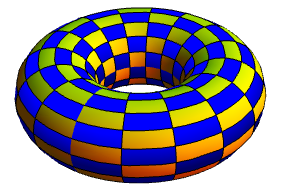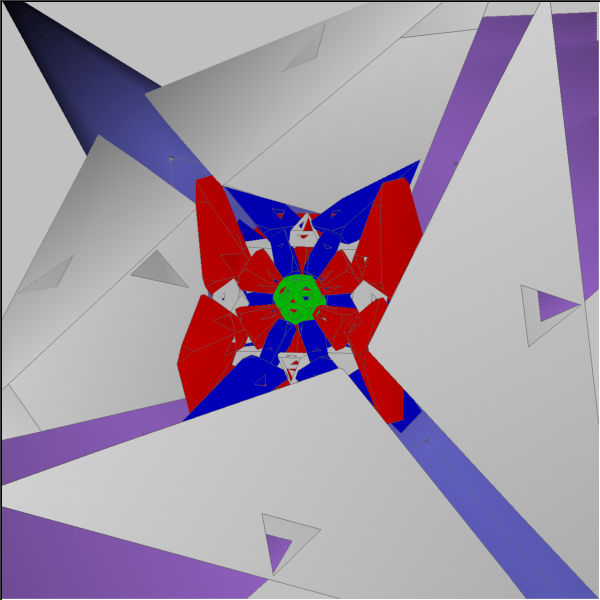# Holyhedra

Euler’s formula famously relates the number of vertices, edges and faces of a polyhedron. Specifically, it gives$V - E + F = 2$, where V, E and F are the numbers of vertices, edges and faces, respectively. For example, the dodecahedron has 20 vertices, 30 edges and 12 faces, and can be easily seen to obey Euler’s formula. A proof is obtained by puncturing one of the faces and ‘unfolding’ it into a planar graph, whence you can proceed by induction on the number of vertices and edges.

It does, however, assume that the polyhedron is homeomorphic to a sphere. We can find a counter-example to Euler’s formula, such as this torus:Here, there are n vertices, n faces and 2n edges. A refinement of Euler’s formula is$V - E + F = \chi$, where$\chi = 2 - 2G$ is the Euler characteristic expressed in terms of the genus (number of holes).

Even this refinement of the formula makes an assumption, namely that none of the faces contain holes. For instance, the following polyhedron (composed of two tetrahedra, one of which penetrates the other) has 7 vertices, 15 edges and 8 faces, but a genus of 0, and therefore disobeys Euler’s formula.John Conway and David Wilson wondered whether or not it would be possible for all faces of a polyhedron to contain holes. They coined the term ‘holyhedron’ to refer to this, even though no explicit examples were known at the time. Two years later, in 1999, Jade Vinson employed a ‘just do it’ construction to synthesise an example. The construction was incredibly fiddly, resulting in a holyhedron with 78585627 faces, which is explained very clearly in Vinson’s paper. John Conway offered a prize of$\frac{10000}{F}$ USD for a holyhedron, so this would be worth 12.7 millicents.

A much more reasonable example is a 492-face beast discovered by Don Hatch. It features many interpenetrating tetrahedra and pentagonal pyramids, based around a central dodecahedron.It was constructed in layers, shown in different colours in the above image. He has included a digraph (directed graph) detailing which layers penetrate the faces of the other layers to achieve the property of being a holyhedron. Eight vertices on the exterior do not penetrate anything; these form the convex hull of the polyhedron.

This entry was posted in Uncategorized. Bookmark the permalink.